# Two vases

Michaela has two vases in her collection. The first vase has the shape of a cone with a base diameter d = 20 cm; the second vase has the shape of a truncated cone with the lower base d1 = 25 cm and with the diameter of the upper base d2 = 15 cm. Which vase can hold more water if the height of both vases is 0.5 m?

V1 =  5235.9878 cm3
V2 =  5104.1667 cm3

### Step-by-step explanation: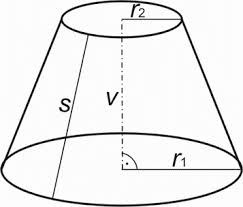Did you find an error or inaccuracy? Feel free to write us. Thank you!Tips to related online calculators
Do you want to convert length units?
Do you know the volume and unit volume, and want to convert volume units?

#### You need to know the following knowledge to solve this word math problem:

We encourage you to watch this tutorial video on this math problem:

## Related math problems and questions:

• Frustum of a cone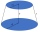A reservoir contains 28.54 m3 of water when full. The diameter of the upper base is 3.5 m, while at the lower base is 2.5 m. Find the height if the reservoir is in the form of a frustum of a right circular cone.
• Wooden bowls20 wooden bowls in the shape of a truncated cone should be painted on the outside and inside with wood varnish. We need 0.1 l of paint to paint 200 cm2. How many liters of paint do we have to buy if the bowls are 25 cm high, the bottom of the bowl has a d
• Truncated cone 5The height of a cone 7 cm and the length of side is 10 cm and the lower radius is 3cm. What could the possible answer for the upper radius of truncated cone?
• Truncated pyramid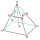The truncated regular quadrilateral pyramid has a volume of 74 cm3, a height v = 6 cm, and an area of the lower base 15 cm2 greater than the upper base's content. Calculate the area of the upper base.
• Runcated pyramid teapot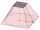The 35 cm high teapot has the shape of a truncated pyramid with the length of the edge of the lower square base a=50 cm and with the edges of the rectangular base b: 20 cm and c: 30 cm. How many liters of water will fit in the teapot?
• Truncated coneA truncated cone has bases with radiuses of 40 cm and 10 cm and a height of 25 cm. Calculate its surface area and volume.
• The cone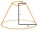The cone with a base radius of 12 cm and a height of 20 cm was truncated at a distance of 6 cm from the base, thus creating a truncated cone. Find the radius of the base of the truncated cone.
• Lamp coneCalculate the surface of a lampshade shaped of a rotary truncated cone with a base diameter of 32 cm and 12 cm and height of 24 cm.
• Octagonal prism vase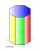0.7 l of water can be poured in an octagonal prism vase. What is the height of the vase, if the bottom has a area of 25 cm square and a thickness of 12 mm?
• Truncated coneCalculate the height of the rotating truncated cone with volume V = 794 cm3 and a base radii r1 = 9.9 cm and r2 = 9.8 cm.
• The truncatedThe truncated rotating cone has bases with radii r1 = 8 cm, r2 = 4 cm and height v = 5 cm. What is the volume of the cone from which the truncated cone originated?
• Rotary coneRotary cone whose height is equal to the circumference of the base, has a volume 229 cm3. Calculate the radius of the base circle and height of the cone.
• Water overflow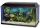A rectangular container that has a length of 30 cm, a width of 20 cm, and a height of 24 cm is filled with water to a depth of 15 cm. When an additional 6.5 liters of water are poured into the container, some water overflows. How many liters of water over
• Truncated pyramidThe concrete pedestal in the shape of a regular quadrilateral truncated pyramid has a height of 12 cm, the pedestal edges have lengths of 2.4 and 1.6 dm. Calculate the surface of the base.
• Truncated cone 3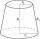The surface of the truncated rotating cone S = 7697 meters square, the substructure diameter is 56m and 42m, find the height of the tang.
• Cylinder horizontallyThe cylinder with a diameter of 3 m and a height/length of 15 m is laid horizontally. Water is poured into it, reaching a height of 60 cm below the axis of the cylinder. How many hectoliters of water is in the cylinder?
• Truncated coneCalculate the volume of a truncated cone with base radiuses r1=13 cm, r2 = 10 cm and height v = 8 cm.